三角形问题_三角形问题等价类测试 - CSDN

• 很不容易找到的资源，希望与大家一起分享。三角形问题是软件测试初学者应该熟悉的经典问题，本人一直苦于测试用例的编写，幸好找到这么好的东东，急于与大家分享。
• 三角形问题：输入三条边是否组成三角行并判断是什么样的三角形。三角形问题
• 输入a,b,c,如果这三个数...然后判断三角形是等腰三角形还是等边三角形，若是等腰三角形则打印输出等腰三角形，若是等边三角形则打印输出等边三角形，否则退出。请给出程序流程及控制流程，测试路径。试举一个测试用例.
问题及描述：
/*
*文件名称：test.cpp
*作者：杨昊
*版本：v6.0
*时间；2016年4月7日

*
*问题描述：三角形问题

*输入描述：无
*程序输出：
*/

#include<iostream>
using namespace std;
class Triangle
{
public:
Triangle(int xx,int yy,int zz)
{
x=xx;
y=yy;
z=zz;
}
void showMessage()
{
cout<<x<<" "<<y<<" "<<z<<endl;
}
private:
int x,y,z;
};

int main()
{
Triangle Tri(7,8,9);
Tri.showMessage();
}

结果及总结：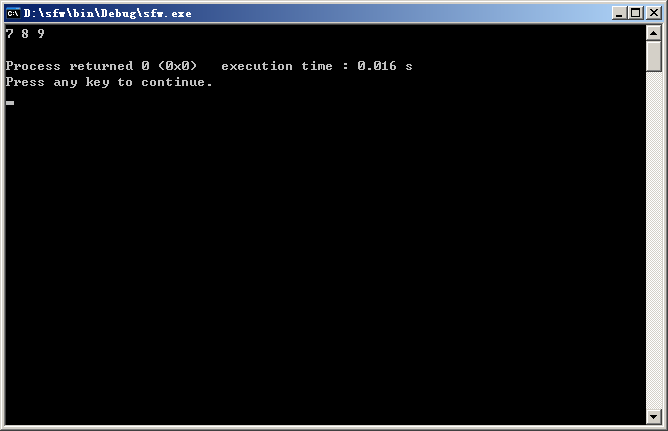展开全文• 参加过大公司面试的读者都清楚，很多公司很喜欢出个算法题，然后评判候选人水平如何，笔者也有过类似经历，接下来是笔者总结的一些常见关于三角形类的算法题。 求面积 理论：除了常见公式S=1/2ah，还有一个不常见的...
参加过大公司面试的读者都清楚，很多公司很喜欢出个算法题，然后评判候选人水平如何，笔者也有过类似经历，接下来是笔者总结的一些常见关于三角形类的算法题。

求面积
理论：除了常见公式S=1/2ah，还有一个不常见的公式海伦公式，S=sqrt(s*(s-a)(s-b)(s-c))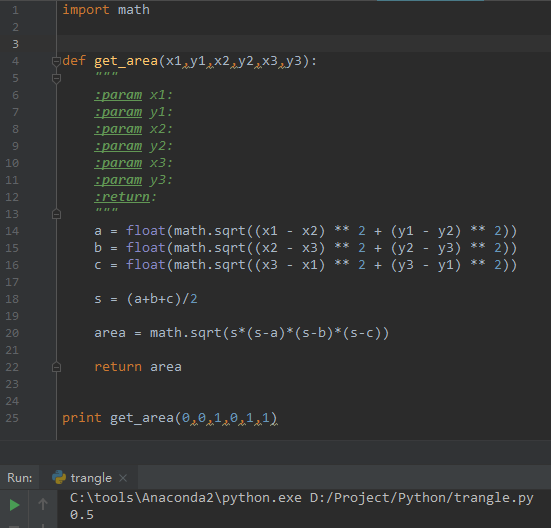判断某个点是否在三角形内部
理论：如果一个点在三角形内，其与三角形的三个点构成的三个子三角形的面积等于大三角形的面积。否则，大于大三角形的面积。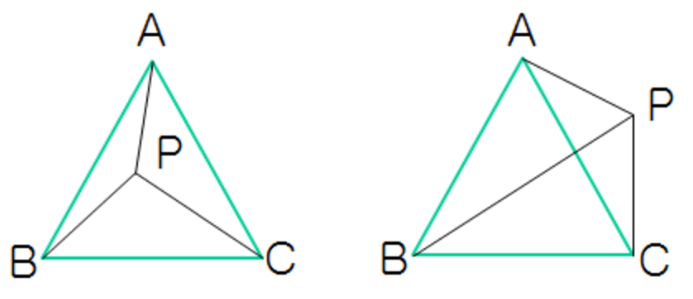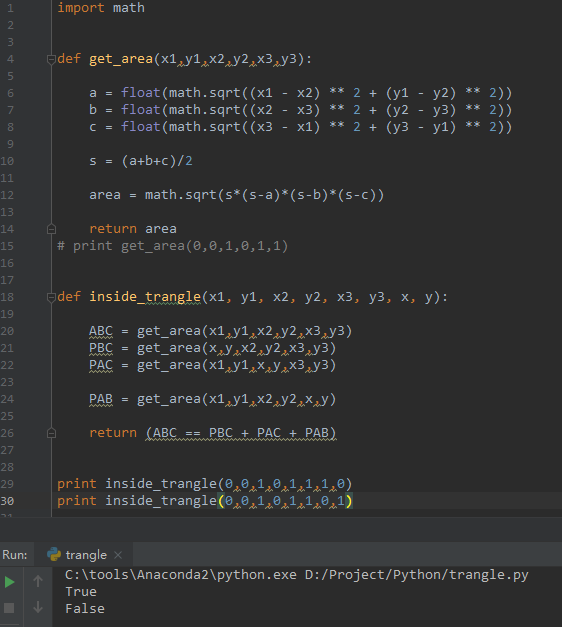参考文献
1.百度百科之海伦公式
2.Algorithm: 如何判断一个点是否在一个三角形内
3.Github项目源代码


展开全文算法 三角形
•  符号三角形第一行有n个符号，符号三角形问题要求对于给定的n，计算有多少个不同的符号三角形，使其所含的“+”和“-”的个数相同。   解题思路：  1、不断改变第一行的每个符号，搜索符合条件的解，可以使用...
问题描述：

下图是由14个“+”和14个“-”组成的符号三角形。2个同号下面都是“+”，2个异号下面都是“-”：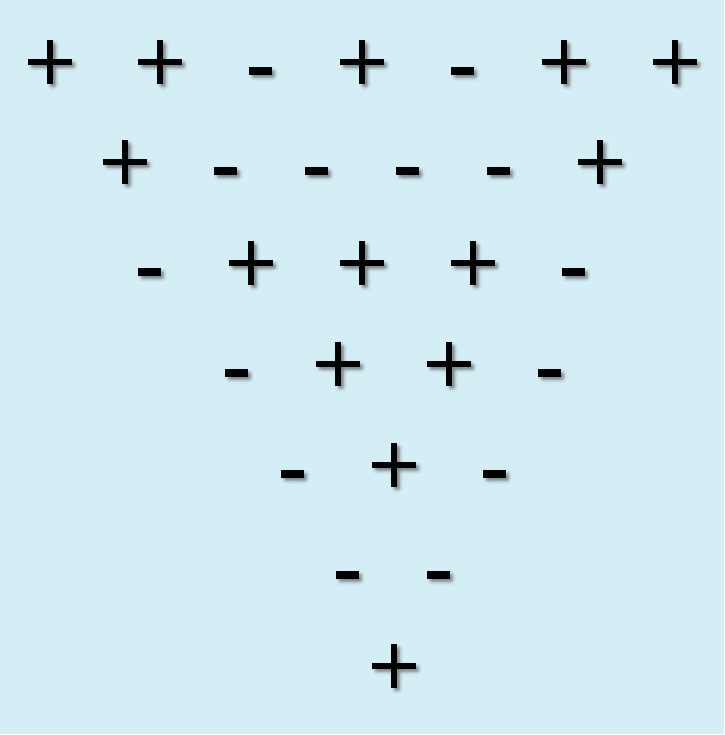符号三角形第一行有n个符号，符号三角形问题要求对于给定的n，计算有多少个不同的符号三角形，使其所含的“+”和“-”的个数相同。

解题思路：

1、不断改变第一行的每个符号，搜索符合条件的解，可以使用递归回溯。

为了便于运算，设+ 为0，- 为1，这样可以使用异或运算符表示符号三角形的关系：
++为+即0^0=0, --为+即1^1=0, +-为-即0^1=1, -+为-即1^0=1

2、因为两种符号个数相同，可以对题解树剪枝

当所有符号总数为奇数时无解，当某种符号超过总数一半时无解

算法设计：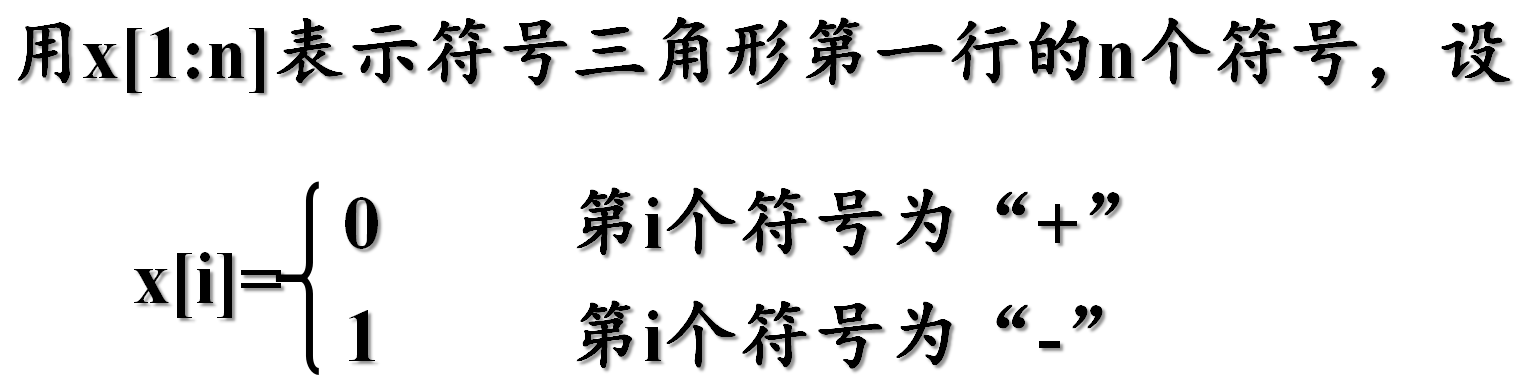在符号三角形的第一行的前i个符号x[1:i]确定后，就确定了一个由i*(i+1)/2个符号组成的符号三角形。

下一步确定了x[1:i]的值后，只要在前面确定的符号三角形的右边加一条边，就可以扩展为x[1:i]所对应的符号三角形。

在回溯法搜索过程中可用当前符号三角形所包含的“+”号个数与“-”号个数均不超过n*(n-1)/4作为可行性约束，用于剪去不满足约束条件的子树。

代码：

需要的数据：

        static int n;//第一行的符号个数
static int half;//n*(n+1)/4
static int count;//当前“+”或者“-”的个数
static int[][] p;//符号三角形矩阵
static long sum;//已找到的符号三角形的个数

初始化及计算：

public static float Compute(int nn) {
n = nn;
count = 0;
sum = 0;
half = (n*(n+1))>>1;
if((half>>1)<<1 != half) {
return 0;
}
half = half>>1;
p = new int[n+1][n+1];
backtrack(1);

return sum;
}

回溯算法1（仅用于计算行数或者列数为n的符号三角形的总数，没有显示符号三角形的功能，但是是核心代码）：

public static void backtrack01(int t) {
if(count>half || (t*(t-1)/2-count > half)) {//对题解树的剪枝
return;
}
if(t>n) {
sum++;//符号三角形的总数目+1
}
else {
//每个位置都有两种情况0,1
for(int i = 0;i<2;i++) {
p[t] = i;
count += i;//对"-"个数进行技术，为了进行剪枝操作

//接下来绘制其余的n-1行
for(int j = 2;j<=t;j++) {
//通过异或的方式求其余行数的放置方式
p[j][t-j+1] = p[j-1][t-j+1]^p[j-1][t-j+2];
count += p[j][t-j+1];
}
backtrack01(t+1);

//恢复现场
for(int j = 2;j<=t;j++) {
count -= p[j][t-j+1];
}
count -= i;
}
}
}

回溯算法2（具备打印符号三角形的功能）：

public static void backtrack(int t) {
if((count>half)||(t*(t-1)/2-count > half)) //对题解树的剪枝
return;
if(t>n) {
sum++;
//打印符号三角形
for(int i =1;i<=n;i++) {
for(int k = 1;k<i;k++) {
System.out.print(" ");
}
for(int j =1;j<=n;j++) {
if(p[i][j] == 0 && j<=n-i+1) {
System.out.print("+" + " ");
}
else if(p[i][j] == 1 && j<=n-i+1) {
System.out.print("-" + " ");
}
else {
System.out.print("  ");
}
}
System.out.println();
}
System.out.println();
}
else {
//每个位置都有两种情况0,1
for(int i =0;i<2;i++) {
p[t] = i;
count += i;//计算“-”的个数

//接下来绘制其余的n-1行
for(int j = 2;j<=t;j++) {
//通过异或的方式求其余行数的放置方式
p[j][t-j+1] = p[j-1][t-j+1]^p[j-1][t-j+2];
count += p[j][t-j+1];

}
backtrack(t+1);

//恢复现场
for(int j =2;j<=t;j++) {
count -= p[j][t-j+1];
}
count -= i;

}
}
}

完整代码：

package tri;

public class TRAN {

static int n;//第一行的符号个数
static int half;//n*(n+1)/4
static int count;//当前“+”或者“-”的个数
static int[][] p;//符号三角形矩阵
static long sum;//已找到的符号三角形的个数

public static float Compute(int nn) {
n = nn;
count = 0;
sum = 0;
half = (n*(n+1))>>1;
if((half>>1)<<1 != half) {
return 0;
}
half = half>>1;
p = new int[n+1][n+1];
backtrack(1);

return sum;
}

/**
* 算法1
* @param t
*/
/*
public static void backtrack01(int t) {
if(count>half || (t*(t-1)/2-count > half) {//对题解树的剪枝
return;
}
if(t>n) {
sum++;//符号三角形的总数目+1
}
else {
//每个位置都有两种情况0,1
for(int i = 0;i<2;i++) {
p[t] = i;
count += i;//对"-"个数进行技术，为了进行剪枝操作

//接下来绘制其余的n-1行
for(int j = 2;j<=t;j++) {
//通过异或的方式求其余行数的放置方式
p[j][t-j+1] = p[j-1][t-j+1]^p[j-1][t-j+2];
count += p[j][t-j+1];
}
backtrack01(t+1);

//恢复现场
for(int j = 2;j<=t;j++) {
count -= p[j][t-j+1];
}
count -= i;
}
}
}
*/

public static void backtrack(int t) {
if((count>half)||((t*(t-1)/2-count > half)) //对题解树的剪枝
return;
if(t>n) {
sum++;
//打印符号三角形
for(int i =1;i<=n;i++) {
for(int k = 1;k<i;k++) {
System.out.print(" ");
}
for(int j =1;j<=n;j++) {
if(p[i][j] == 0 && j<=n-i+1) {
System.out.print("+" + " ");
}
else if(p[i][j] == 1 && j<=n-i+1) {
System.out.print("-" + " ");
}
else {
System.out.print("  ");
}
}
System.out.println();
}
System.out.println();
}
else {
//每个位置都有两种情况0,1
for(int i =0;i<2;i++) {
p[t] = i;
count += i;//计算“-”的个数

//接下来绘制其余的n-1行
for(int j = 2;j<=t;j++) {
//通过异或的方式求其余行数的放置方式
p[j][t-j+1] = p[j-1][t-j+1]^p[j-1][t-j+2];
count += p[j][t-j+1];

}
backtrack(t+1);

//恢复现场
for(int j =2;j<=t;j++) {
count -= p[j][t-j+1];
}
count -= i;

}
}
}

public static void main(String[] args) {
// TODO Auto-generated method stub
float SUM = Compute(4);
System.out.print("总数： " + SUM);
}

}


以行数为4的符号三角形为例，显示输出如下：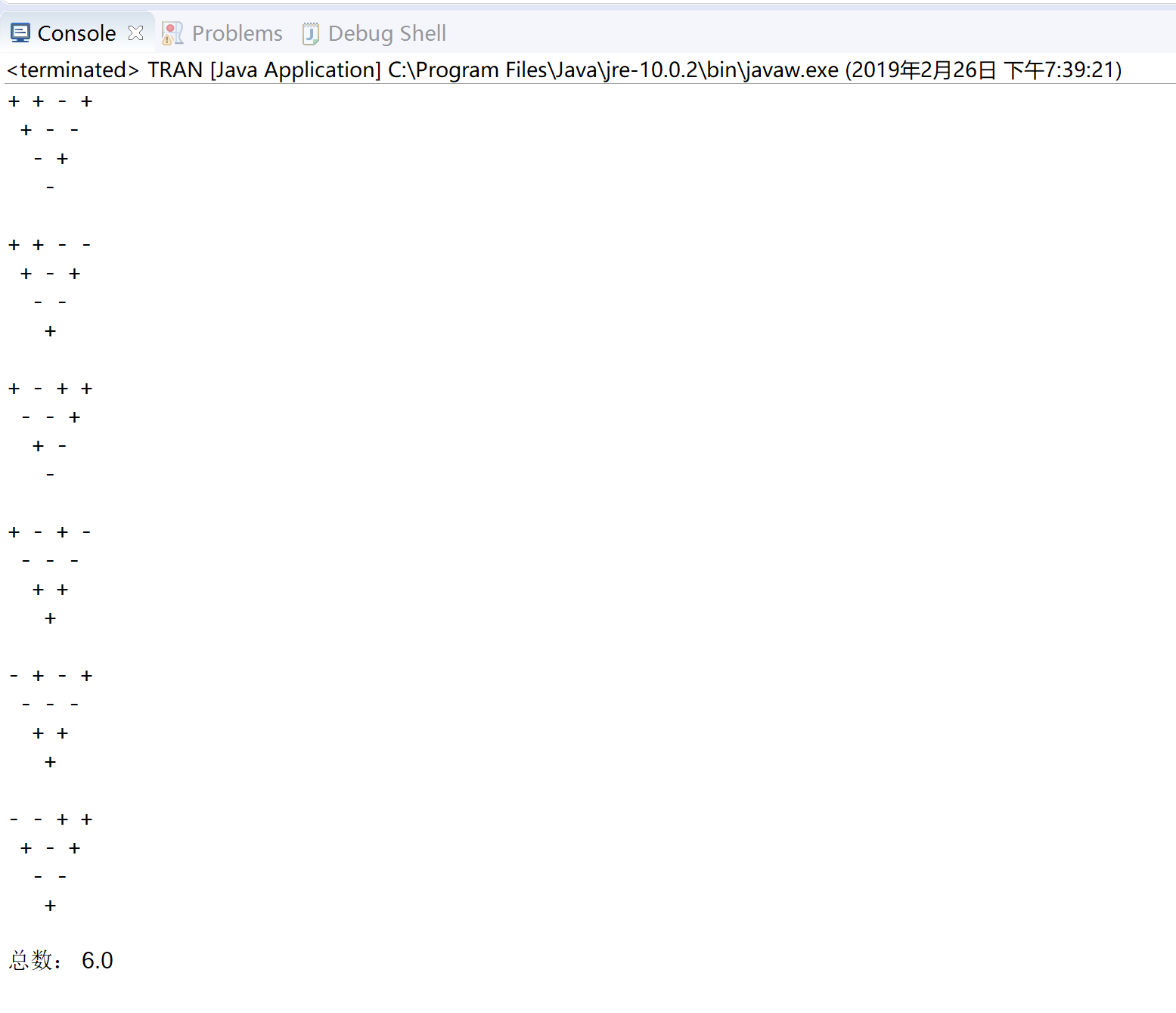展开全文回溯算法
• 白盒测试 预习报告 一、实验目的 1.掌握覆盖率的计算方法 2.掌握语句覆盖，分支覆盖（判定覆盖），条件覆盖，条件分支组合覆盖，组合覆盖的含义及区别 3.掌握使用各种覆盖法，设计测试用例的方法 ...
白盒测试
预习报告
一、实验目的
1.掌握覆盖率的计算方法
2.掌握语句覆盖，分支覆盖（判定覆盖），条件覆盖，条件分支组合覆盖，组合覆盖的含义及区别
3.掌握使用各种覆盖法，设计测试用例的方法
二、实验原理
1 测试覆盖率
测试覆盖率是指用于确定测试所执行到的覆盖项的百分比。其中的覆盖项是指作为测试基础的一个入口或属 性，比如语句、分支、条件等。 测试覆盖率可以表示出测试的充分性，在测试分析报告中可以作为量化指标的依据，测试覆盖率越高效果越 好。但覆盖率不是目标，只是一种手段。测试覆盖率包括功能点覆盖率和结构覆盖率。 功能点覆盖率大致用于表示软件已经实现的功能与软件需要实现的功能之间的比例关系。 结构覆盖率包括语句覆盖率、分支覆盖率、循环覆盖率、路径覆盖率等。
(1)语句覆盖率
语句覆盖率=至少被执行一次的语句数量/可执行的语句总数100%
(2)判定覆盖率
判定覆盖率=判定结果被评价的次数/判定结果的总数100%
(3)条件覆盖率
条件覆盖率=条件操作数值至少被评价一次的数量/条件操作数值的总数*100%
(4)判定/条件覆盖率
判定/条件覆盖率=条件操作数值或判定结果值至少被评价一次的数量/（条件操作数值总数+判定结果总数） 100%
(5)条件组合覆盖率
条件组合覆盖率=条件操作数值至少被评价一次的数量/条件操作数值的所有组合总数100%
2 逻辑覆盖
根据覆盖目标的不同和覆盖源程序语句的详尽程度，逻辑覆盖又可分为语句覆盖、判定覆盖、条件覆盖、条件 判定组合覆盖、多条件覆盖（条件组合覆盖）、组合覆盖。
(1)语句覆盖
语句覆盖是选择足够多的测试数据，使得程序中的每个可执行语句至少执行一次。语句覆盖的缺点是对程序执 行逻辑的覆盖率很低。 例如：图3-1为一个程序流程图，它的源程序是：
PROCEDURE M(VAR A，B，X：REAL)；
BEGIN IF(A>1) AND (B=0) THEN X:=X/A；
IF(A=2) OR (X>1) THEN X:=X+1；
END
为使程序中每个语句至少执行一次，只需设计一个能通过路径ace的例子就可以了，例如选择输入数据为： A=2，B=0，X=3，就可达到“语句覆盖”标准。
(2)判定覆盖
判定覆盖是通过设计足够多的测试用例，使得程序中的每一个判定至少获得一次真值和假值，或者使得程序中 的每一个取真的分支或取假的分支至少经历一次，也称为分支覆盖。判定覆盖的缺点是主要对整个表达式的最终取 值进行度量，忽略了表达式的内部取值。对图3-1的程序，如果设计两个用例，使它们能通过路径ace和abd，或者 通过路径acd和abe，就可达到“判定覆盖”标准，为此，可以选择输入数据为：
① A=3，B=0，X=1(沿路径acd执行)；
② A=2，B=1，X=3(沿路径abe执行)。
(3)条件覆盖
条件覆盖是通过设计足够多的测试用例，使得程序中每个判定包含的每个条件的可能取值（真/假）都至少满 足一次。条件覆盖的缺点是不能够满足判定覆盖。 图3-1的程序有四个条件：A＞1、B=0、A=2、X＞1
为了达到“条件覆盖”标准，需要执行足够的测试用例使得在a点有：A＞1、A≤1、B=0、B≠0等各种结果出 现，以及在b点有：A=2、A≠2、X＞1、X≤1等各种结果出现。现在只需设计以下两个测试用例就可满足这一标准：
① A=2，B=0，X=4（沿路径ace执行）；
② A=1，B=1，X=1（沿路径abd执行）。
(4)条件判定组合覆盖
条件判定组合覆盖（判定/条件覆盖）是通过设计足够多的测试用例，使得程序中每个判定包含的每个条件的 所有情况（真/假）至少出现一次，并且每个判定本身的判定结果（真/假）也至少出现一次。条件判定组合覆盖的测试用例一定同时满足判定覆盖和条件覆盖。条件判定组合覆盖的缺点是没有考虑单个判定对整体结果的影响，无 法发现逻辑错误。对图3-1的程序，可以设计两个测试用例满足条件判定组合覆盖：
① A=2，B=0，X=4(沿路径ace执行)；
② A=1，B=1，X=1 (沿路径abd执行)。
(5)条件组合覆盖
条件组合覆盖也称多条件覆盖，是通过设计足够多的测试用例，使得每个判定中条件的各种可能组合都至少出 现一次（以数轴形式划分区域，提取交集，建立最少的测试用例）。条件组合覆盖的缺点是判定语句较多时，条件 组合值比较多。
再看图3-1的程序，我们需要选择适当的例子，使得下面8种条件组合都能够出现：
① A>1，B=0
② A>1，B≠0
③ A≤1，B=0
④ A≤1，B≠0
⑤ A=2，X>1
⑥ A=2，X≤1
⑦ A≠2，X>1
⑧ A≠2，X≤1
必须注意到，⑤、⑥、⑦、⑧四种情况是第二个IF语句的条件组合，而X的值在该语句之前是要经过计算的， 所以还必须根据程序的逻辑推算出在程序的入口点X的输入值应是什么。
下面四个例子可以使上述8种条件组合至少出现一次：
A=2，B=0，X=4使①、⑤两种情况出现；
A=2，B=1，X=1使②、⑥两种情况出现；
A=1，B=0，X=2使③、⑦两种情况出现；
A=1，B=1，X=1使④、⑧两种情况出现。
(6)组合覆盖
组合覆盖是通过执行足够多的测试用例，使得程序中每个判定的所有可能的条件取值组合都至少出现一次。满 足组合覆盖的测试用例一定满足判定覆盖、条件覆盖和条件判定组合覆盖。
实验报告
一、实验题目
白盒测试—逻辑覆盖—三角形问题
二、实验内容及结果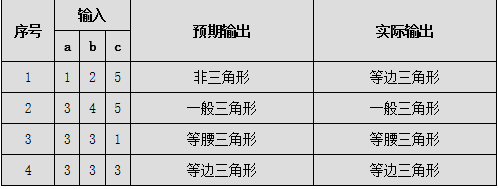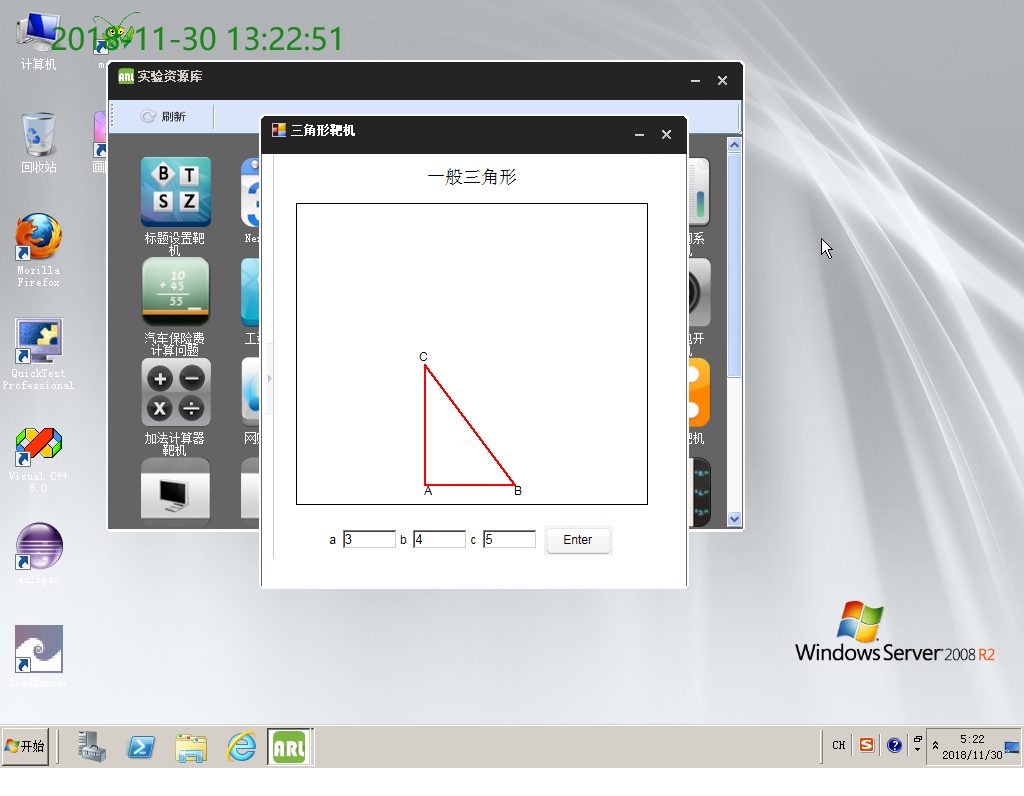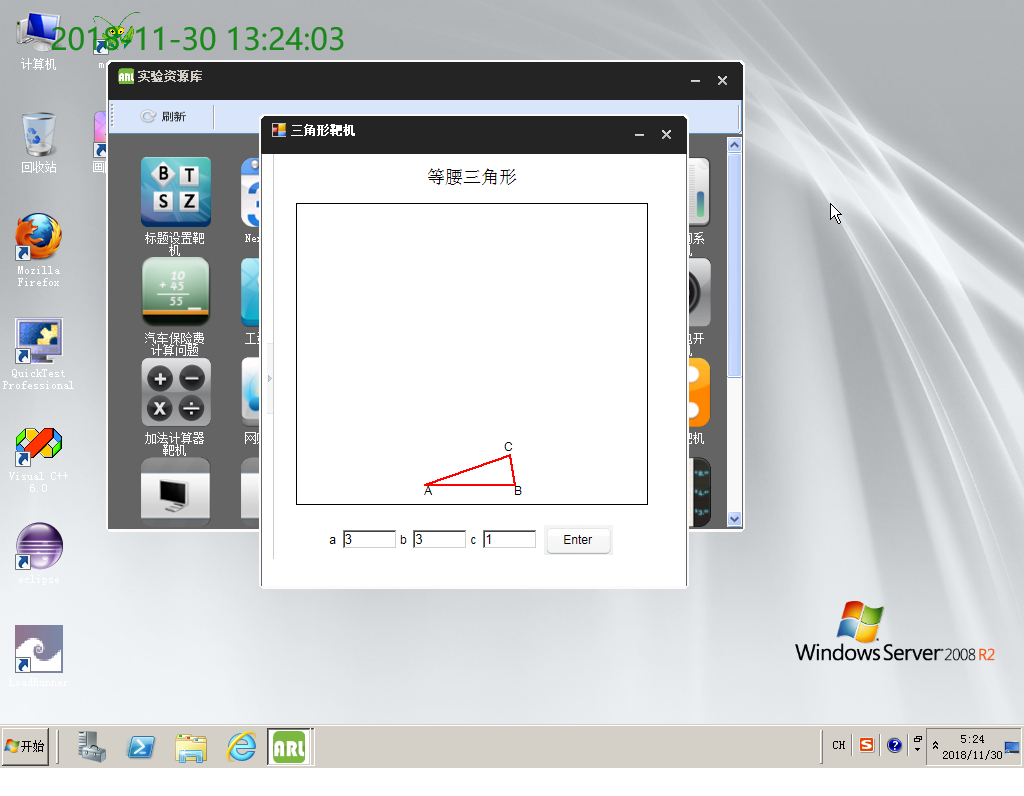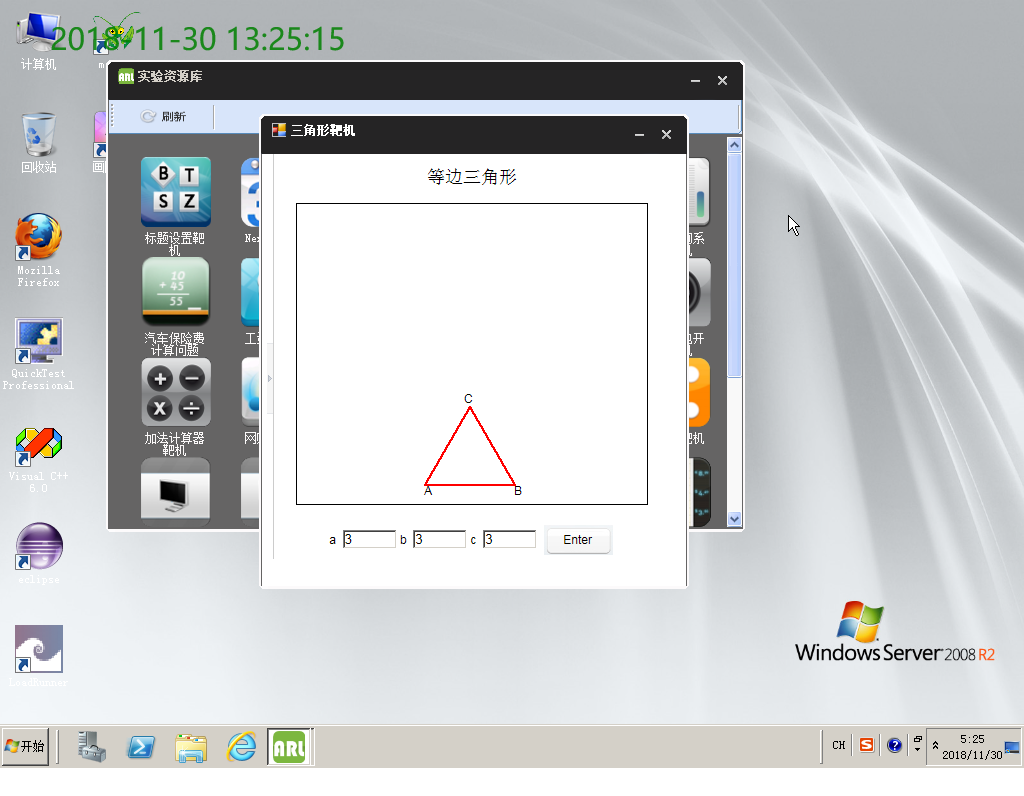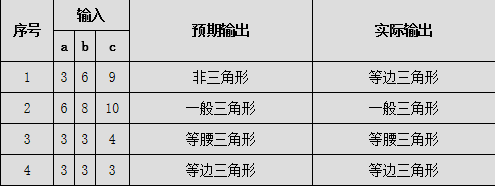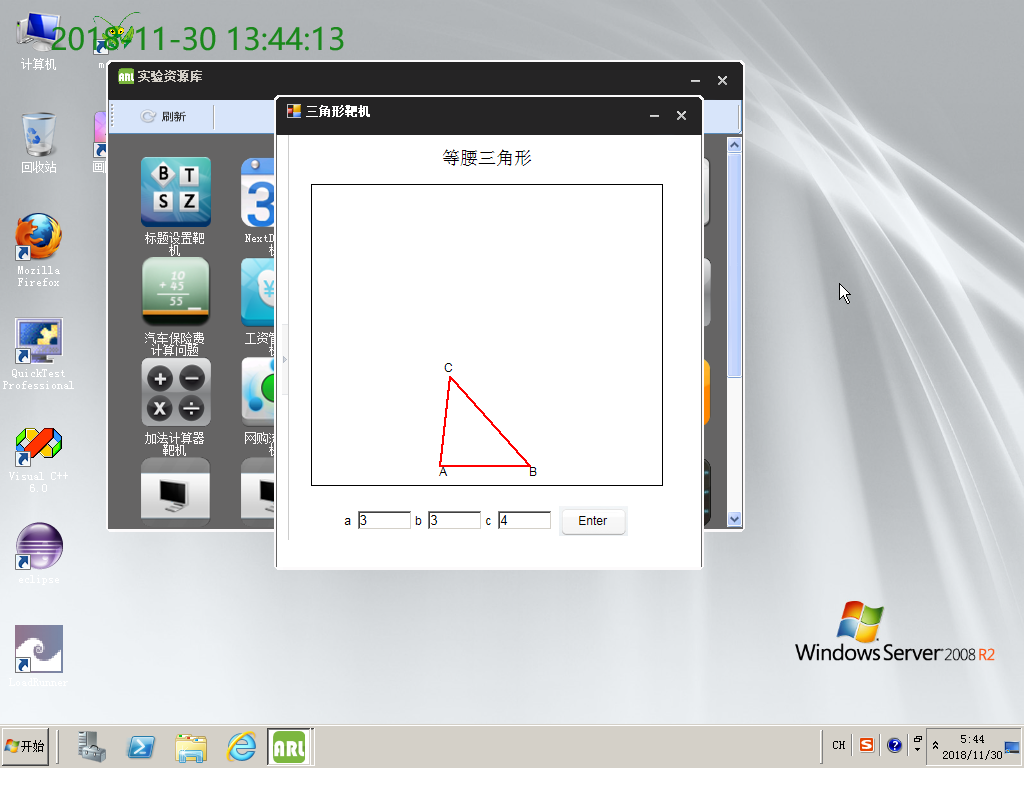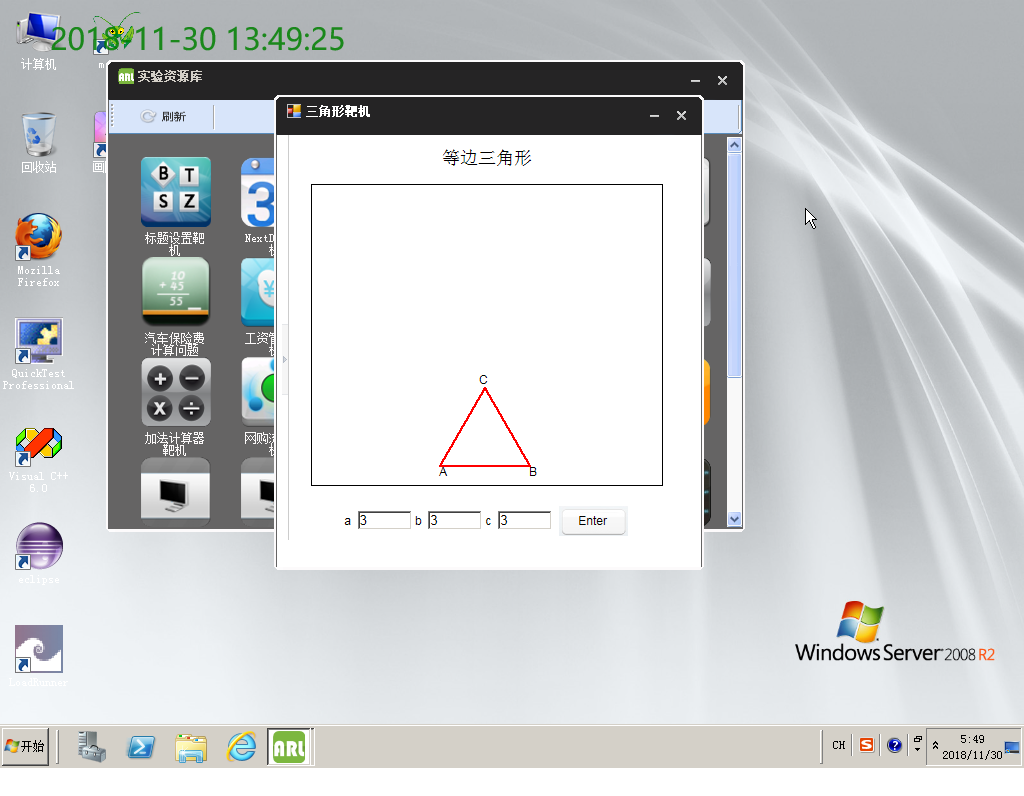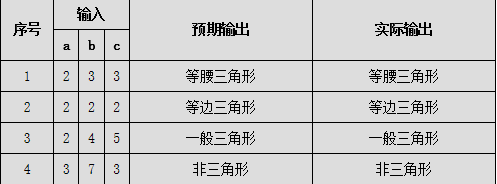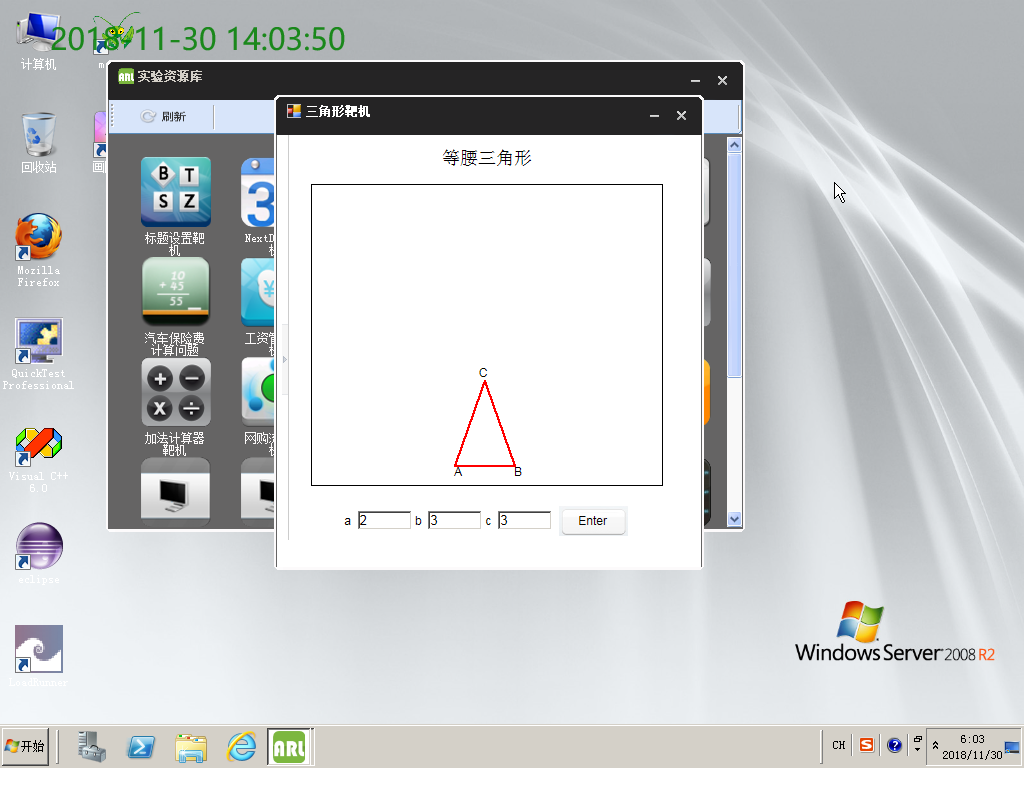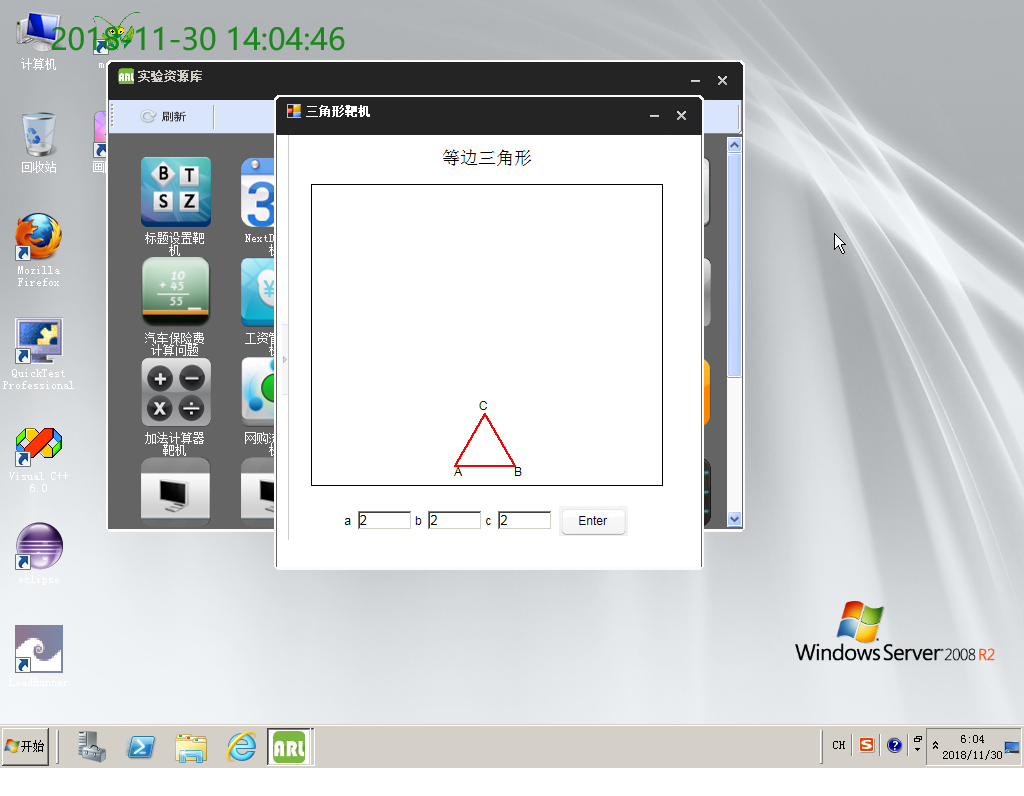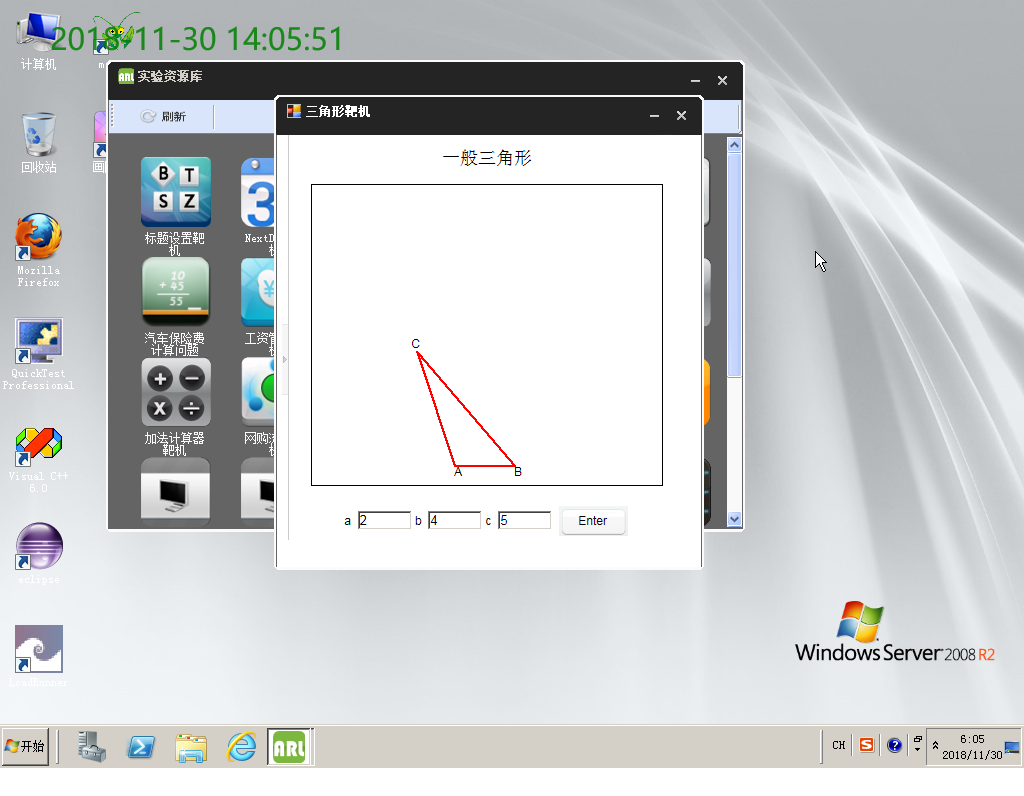展开全文软件工程 白盒测试
• 问题描述：给定由一个n行数字组成的数字三角形，计算从三角形的顶至底的路径经过的数字和的最大值。 C语言代码如下： #include<stdio.h> #include<stdlib.h> #define MAXSIZE 10 int MaxTriangle(int v...
• B——数字三角形问题 题目 #include<stdio.h> // 动态规划法求: 数字三角形—1730 int main() { int n,i,j,D, a; scanf("%d",&n); for (i=1; i<=n; i++) // 输入三角形 for(j=...
• 有一个由非负整数组成的三角形，第一行只有一个数，除了最下行之外每个数的左下方和右下方各有一个数.  1  3 2  4 10 1 4 3 2 20 从第一行的数开始，每次可以往左下或右下走一格，直到走到最下行，把沿途...算法
• 数字三角形问题详解 题目描述 给定一个如下图所示的数字三角形，从顶部出发，在每一结点可以选择移动至其左下方的结点或移动至其右下方的结点，一直走到底层，要求找出一条路径，使路径上的数字的和最大。算法 DP 动态规划 数字三角形
• ## Python判断三角形

万次阅读 2017-09-13 15:57:06
# All rightsreserved ...#问题分析：组成三角形的条件是任意两边之和大于第三边，如果条件成立，则能构成三角形。条件表达式中的多个条件必须全部成立，条件之间使用and运算符连接。代码如下： sid
• ## 三角形测试用例

万次阅读 2016-01-29 10:56:16
三角形计算中，要求三角形的三个边:ABC。 1.当三边不可能构成三角形时提示错误，可构成三角形时计算三角形周长。 2.若是等腰三角形打印“等腰三角形”，若两个等腰的平方和等于第三边平方和，则打印“等腰直角...三角形测试用例
• 案例实战—直角三角形试用等价类划分、边界值分析和因果图加判定表驱动法为三角形问题中的直角三角形设计测试用例。假定三边边长a、b和c均为1到100之间的正整数，其中整数的要求通过数据类型控制。直角三角形问题...软件测试
• #问题描述：编写程序，使用双循环输出三角形图案。 #问题分析：本程序由图形知，包含5行，即外层循环执行5次，每行内容的由三部分内容组成：空格、星号、回车，分别通过两个for循环和一条print语句实现。代码如下: ...
• ## 运用Python的turtle库绘制等边三角形

万次阅读 多人点赞 2019-07-20 15:07:30
我最先考虑以最简洁的方式来解决这个问题，也就是以最少代码来完成这个作业并且还要正确。 题目重点：运算turtle库里面的turtle.fd()和turtle.seth()函数，绘制等边三角形。 思路：fd控制长度，而seth则为方向...
• 任意输入3个整数作为三角形的3条边的长度，判断三角形的类型： 第一步：划分有效等价类和无效等价类 输入条件 有效等价类 无效等价类 是否能构成三角形的三条边 a>0 (1) a...等价类划分 三角形
• 问题：根据输入的三角形的三条边判断三角形的类型，并输出他的面积和类型。思路先行：第一步：先判断输入的三条边能否构成三角形。（a+b&gt;c &amp;&amp; a+c&gt;b &amp;&amp; b+c&gt;a...c语言 新手
• 白盒测试实例 1~12： http://blog.csdn.net/aidisheng/article/details/2868709
...